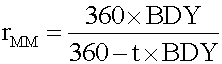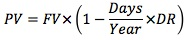Fixed Income I

Reading 44. Introduction to Fixed-Income Valuation

Learning Outcome Statements

g. calculate and interpret yield measures for fixed-rate bonds and floating-rate notes;

h. calculate and interpret yield measures for money market instruments;

CFA Curriculum, 2020, Volume 5

### Why should I choose AnalystNotes?

Simply put: AnalystNotes offers the best value and the best product available to help you pass your exams.

### Subject 6. Yield Measures for Floating-Rate Notes and Money Market Instruments

Floating-Rate Notes

Interest rate volatility affects the price of a fixed-rate bonds. A floating-rate note (a floater, or an FRN) maintains a more stable price than a fixed-rate note because interest payments adjust for changes in market interest rates. With a floater, interest rate volatility affects future interest payments.

The quoted margin is typically the specified yield spread over or under the reference rate, which is often LIBOR. It is used for compensating the investor for the difference in the credit risk of the issuer and that implied by the reference rate.

The discount margin, also known as the required margin, is the spread required by investors and to which the quoted margin must be set in order for the FRN to trade at par value on a rate reset date. Changes in the discount margin usually come from changes in the issuer's credit risk.

Money Market Instruments

Unique characteristics:

• Yield measures are annualized but not compounded.
• Often quoted using non-standard interest rates (discount rate or add-on rate? 360-day year or 365-day year? redemption value amount (FV) or price at issuance (PV)?)
• Different periodicities

Money market instruments need to be converted to a common basis for analysis.

Money market yield (also known as CD equivalent yield) is the annualized HPY on the basis of a 360-day year using simple interest.Discount rate:Note that the denominator is FV, not PV. The rate of return is therefore understated.Note the only difference: the denominator is PV, not FV.

Bond equivalent yield: money market rate stated on a 365-day add-on rate basis.

Example

90-day T-bill, face value 100, quoted discount rate: 2.5% for an assumed 360-day year.

PV = 100 x (1 - 90/360 x 0.025) = 99.375
To calculate the bond equivalent yield for a 365-day year:
AOR = (365/90) x (100 - 99.375)/99.375 = 2.55%

###### DannyM

Do we need to memorize equation 8, the valuation of an FRN?

###### cfa1980

I doubt it. It's important to understand the relationship, not memorizing the formula.

###### robertdole

CFAI notebook has questions on FRN valuation so I imagine you would need to know how to apply the formula

###### khalifa92

LOOOL this equation 8 is so simple it makes a person confidence sky rocket to the moon.

###### ginarapp1

Can you calculate these with BAI II?